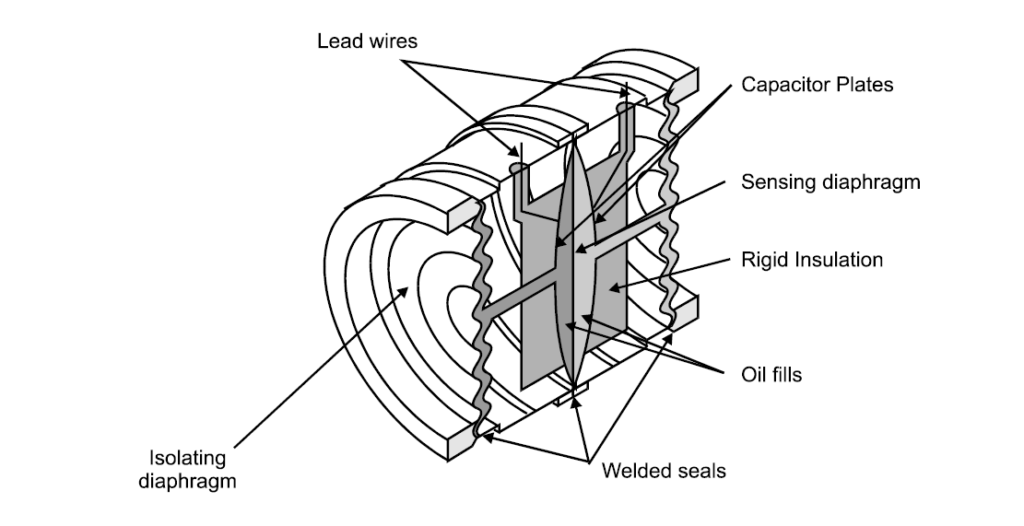# Capacitance pressure transmitterCapacitance pressure transmitter is a pressure measurement device which convert applied pressure into a current signal. Capacitive type pressure transmitter is differential pressure type sensor.

## Principle:

Capacitive pressure transmitter works under the principle of differential capacitance. Capacitive pressure measurement involves sensing the change in capacitance that results from the movement of a diaphragm.Generaly the capacitance is defined by the equation:

C = Aε/d

C – Capacitance between two connductor

A – Area of overlapping between those conductors

d – Distance seperating the conductors

ε – Dielectric permitivity of insulating medium

Permitivity of the medium and the area of overlapping will be constant in this case, the only varying parameter in this case is the distance between the conductors which varies when the pressure varies, which changes the capacitance. So the pressure variation results in the capacitance variation.

## Design:

Two designs are quite common. The first is the two-plate design and is configured to operate in the balanced or unbalanced mode. The other is a single capacitor design.In two plate design the one plate made fixed and other one is kept movable. The varying process pressure is applied to the movable plate and the plate can be kept fixed by applying constant pressure. There are balanced and unbalanced modes in capacitance pressure measurement. The balanced mode is where the reference capacitor is varied to give zero voltage on the output. The unbalanced mode requires measuring the ratio of output to excitation
voltage to determine pressure

A Rosemount capacitance pressure sensor is shown below:The capacitance chamber is isolated from the process with a isolation chamber. The pressure applied at the one side, as pressure at the high pressure side increases the isolating diaphragm gets pushed toward the metal frame, transferring its motion to the sensing diaphragm via the fill fluid. The fill fluid will be oil.

A capacitance detector circuit connected to this cell uses a high-frequency AC excitation signal to measure the different in capacitance between the two halves, translating that into a DC signal which ultimately becomes the signal output by the instrument representing pressure.

The simple capacitance detector connection with the electrical circuit is shown below:• Inaccuracy 0.01 to 0.2%
• Linearity
• Fast response
• Range of 80Pa to 35MPa